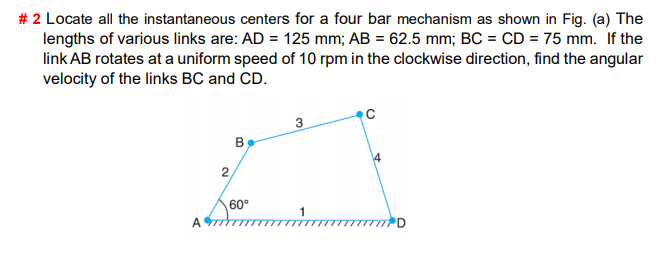# Locate all the instantaneous centers for a four bar mechanism as shown in Fig. (a) The lengths of various links are: AD = 125 mm; AB = 62.5 mm; BC = CD = 75 mm. If the link AB rotates at a uniform speed of 10 rpm in the clockwise direction, find the angular velocity of the links BC and CDs-at-a-uniform-spe

Question-AnswerCategory: Theory Of MachinesLocate all the instantaneous centers for a four bar mechanism as shown in Fig. (a) The lengths of various links are: AD = 125 mm; AB = 62.5 mm; BC = CD = 75 mm. If the link AB rotates at a uniform speed of 10 rpm in the clockwise direction, find the angular velocity of the links BC and CDs-at-a-uniform-spe

Locate all the instantaneous centers for a four bar mechanism as shown in Fig. (a) The lengths of various links are: AD = 125 mm; AB = 62.5 mm; BC = CD = 75 mm. If the link AB rotates at a uniform speed of 10 rpm in the clockwise direction, find the angular velocity of the links BC and CD.Solution:

Solution:

————————————————————————————————————–

Given:

■ Length of AD = 125 mm = 0.125 m

■ Length of AB = 62.5 mm = 0.0625 m

■ Length of BC = CD = 75 mm = 0.075 m

■ Speed of link AB, NAB = 10 rpm

■ Angular velocity at link AB ,ωAB = (2πN) / 60 = (2 x π x 10) / 60 =1.0471 rad/s

————————————————————————————————————–

Objective:

Find the Angular velocities of

————————————————————————————————————–

Step 1 of 4 │Determine the number of Instantaneous centers

The given four bar mechanism have four links (i.e n=4), Therefore number of Instantaneous centers is given byTherefore Six instantaneous centers are in the given four bar mechanics. They are

I12, I14are the fixed instantaneous centres and I13, I23, I24, I34are varying instantaneous centres (Changes with configuration of links)

————————————————————————————————————–

Step 2 of 4 │Locate the instantaneous centres on the figure

Draw the Fourbar mechanism (Refer Figure.1) as per the dimension and locate the instantaneous centres. Measure the distance of the instantaneous from the joints.

Figure.1————————————————————————————————————–

Step 3 of 4 │Determine the angular velocity of the link BC

We know thatTherefore velocity at point B in link AB is given bySubstituting known valuesSince the point B is also a point on link BC, therefore velocity of point B on link BC isFrom the Figure.1,

The distance I13.B =103.83 mm = 0.1038 m

Therefore————————————————————————————————————–

Step 4 of 4 │Determine the angular velocity of the link BC

The instantaneous centre I13 is common for joints B and C, Therefore we can writeFrom the Figure.1,

The distance I13.B =103.83 mm = 0.1038 m
The distance I13.C =75 mm = 0.075 m

Substituting valuesWe know thatTherefore————————————————————————————————————–